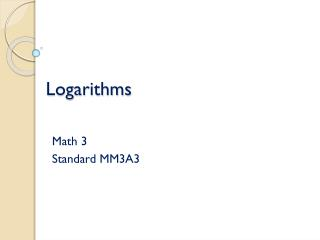Download PresentationLogarithms# Logarithms - PowerPoint PPT Presentation

Download Presentation##### Logarithms

Download Policy: Content on the Website is provided to you AS IS for your information and personal use and may not be sold / licensed / shared on other websites without getting consent from its author. While downloading, if for some reason you are not able to download a presentation, the publisher may have deleted the file from their server.

- - - - - - - - - - - - - - - - - - - - - - - - - - - E N D - - - - - - - - - - - - - - - - - - - - - - - - - - -
##### Presentation Transcript

1. Logarithms Math 3 Standard MM3A3

2. Background: • Before there were calculators that could evaluate powers, mathematicians had to use logarithms • Logarithms are helpful especially when there are non-integer powers • For example:

3. Definition: • The logarithm of x with base a • is denoted as: • This is read “log base a of x” • and defined as:

4. Logarithmic form: Exponential form: Translate from logarithmic form to exponential form:

5. Logarithmic form: Exponential form: Translate from exponential form to logarithmic form:

6. Special Logarithms because because because

7. Evaluate each logarithm

8. Properties of Logarithms Math 3 MM3A2

9. The Product Property • Example:

10. The Quotient Property • Example:

11. The Power Property • Example:

12. Expansion • The properties are used to expand the logarithm • Each factor will have it’s own log • Example: Expand each logarithm

13. Expansion • Example: Expand each logarithm

14. You Try! • Expand the following logarithms using the properties of logarithms:

15. Condense • The properties are used to condense the logarithm • There will be one single log • Example: Condense each logarithm

16. Condense • Example: Condense each logarithm

17. You Try! • Condense the following logarithms in to a single logarithm using the properties of logarithms: